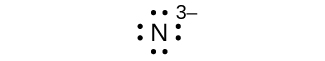# 3.3.1.0: Lewis Dot Diagrams (Problems)

$$\newcommand{\vecs}{\overset { \rightharpoonup} {\mathbf{#1}} }$$ $$\newcommand{\vecd}{\overset{-\!-\!\rightharpoonup}{\vphantom{a}\smash {#1}}}$$$$\newcommand{\id}{\mathrm{id}}$$ $$\newcommand{\Span}{\mathrm{span}}$$ $$\newcommand{\kernel}{\mathrm{null}\,}$$ $$\newcommand{\range}{\mathrm{range}\,}$$ $$\newcommand{\RealPart}{\mathrm{Re}}$$ $$\newcommand{\ImaginaryPart}{\mathrm{Im}}$$ $$\newcommand{\Argument}{\mathrm{Arg}}$$ $$\newcommand{\norm}{\| #1 \|}$$ $$\newcommand{\inner}{\langle #1, #2 \rangle}$$ $$\newcommand{\Span}{\mathrm{span}}$$ $$\newcommand{\id}{\mathrm{id}}$$ $$\newcommand{\Span}{\mathrm{span}}$$ $$\newcommand{\kernel}{\mathrm{null}\,}$$ $$\newcommand{\range}{\mathrm{range}\,}$$ $$\newcommand{\RealPart}{\mathrm{Re}}$$ $$\newcommand{\ImaginaryPart}{\mathrm{Im}}$$ $$\newcommand{\Argument}{\mathrm{Arg}}$$ $$\newcommand{\norm}{\| #1 \|}$$ $$\newcommand{\inner}{\langle #1, #2 \rangle}$$ $$\newcommand{\Span}{\mathrm{span}}$$$$\newcommand{\AA}{\unicode[.8,0]{x212B}}$$

PROBLEM $$\PageIndex{1}$$

Write the Lewis symbols for each of the following ions:

1. As3–
2. I
3. Be2+
4. O2–
5. Ga3+
6. Li+
7. N3–

eight electrons:eight electrons:no electrons

Be2+

eight electrons:no electrons

Ga3+

no electrons

Li+

eight electrons:PROBLEM $$\PageIndex{2}$$

Write the Lewis symbols for the following elements:

1. Cl
2. Na
3. Mg
4. Ca
5. K
6. Br
7. Sr
8. FPROBLEM $$\PageIndex{3}$$

Write the Lewis symbols of the ions in each of the following ionic compounds and the Lewis symbols of the atom from which they are formed:

a. MgS
b. Al2O3
c. GaCl3
d. K2O
e. Li3N
f. KFPROBLEM $$\PageIndex{4}$$

In the Lewis structures listed here, M and X represent various elements in the third period of the periodic table. Write the formula of each compound using the chemical symbols of each element:

(a)(b)(c)(d)MgS

AlCl3

Na2S

Al2S3

PROBLEM $$\PageIndex{5}$$

Identify the atoms that correspond to each of the following electron configurations.

a. 1s22s22p5
b. 1s22s22p63s2
c. 1s22s22p63s23p64s23d104p4
d. 1s22s22p63s23p64s23d104p1

F

Mg

Se

Ga